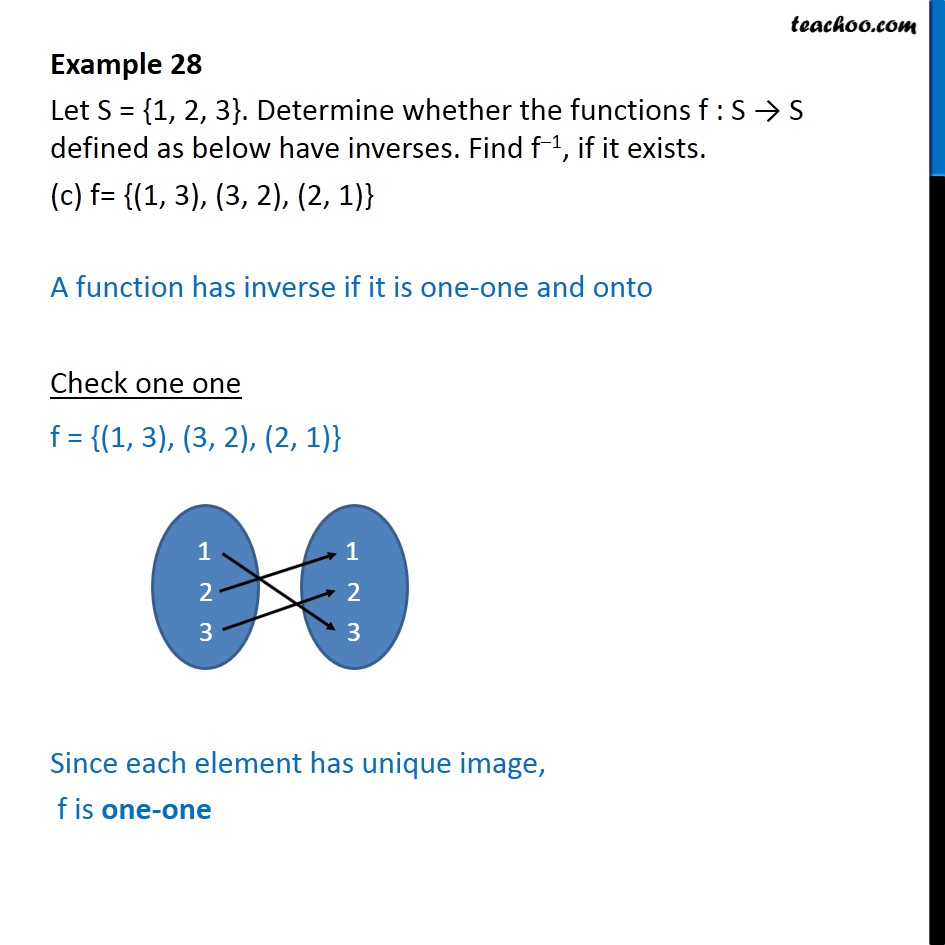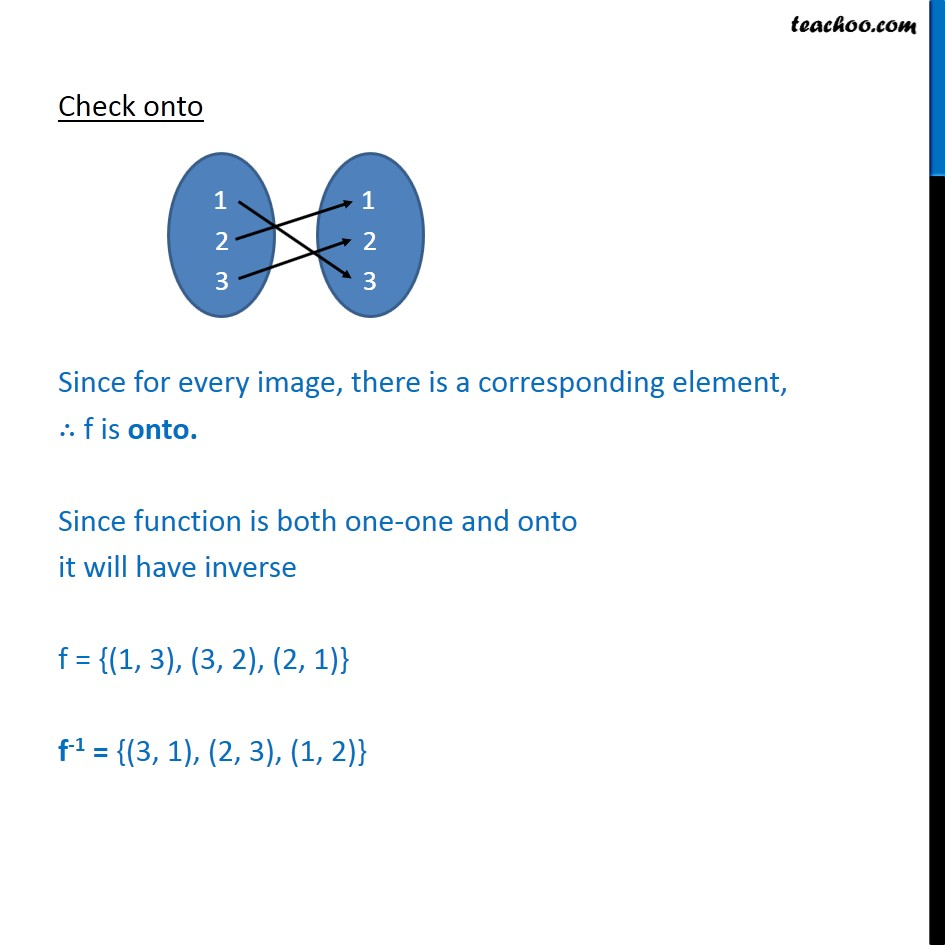Examples

Chapter 1 Class 12 Relation and Functions
Serial order wiseLearn in your speed, with individual attention - Teachoo Maths 1-on-1 Class

### Transcript

Example 28 Let S = {1, 2, 3}. Determine whether the functions f : S → S defined as below have inverses. Find f–1, if it exists. (c) f = {(1, 3), (3, 2), (2, 1)} A function has inverse if it is one-one and onto Check one one f = {(1, 3), (3, 2), (2, 1)} Since each element has unique image, f is one-one Check onto Since for every image, there is a corresponding element, ∴ f is onto. Since function is both one-one and onto it will have inverse f = {(1, 3), (3, 2), (2, 1)} f-1 = {(3, 1), (2, 3), (1, 2)}